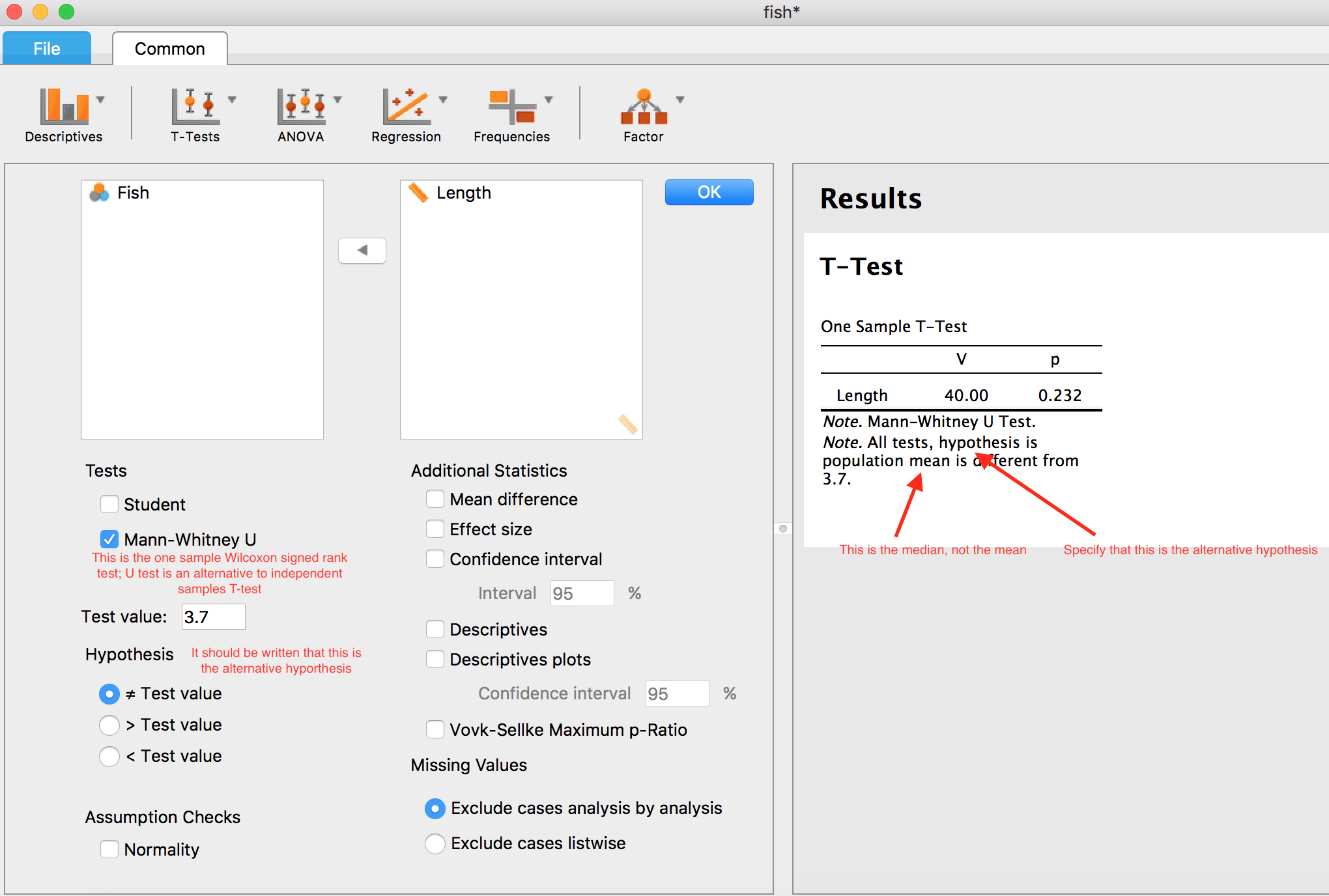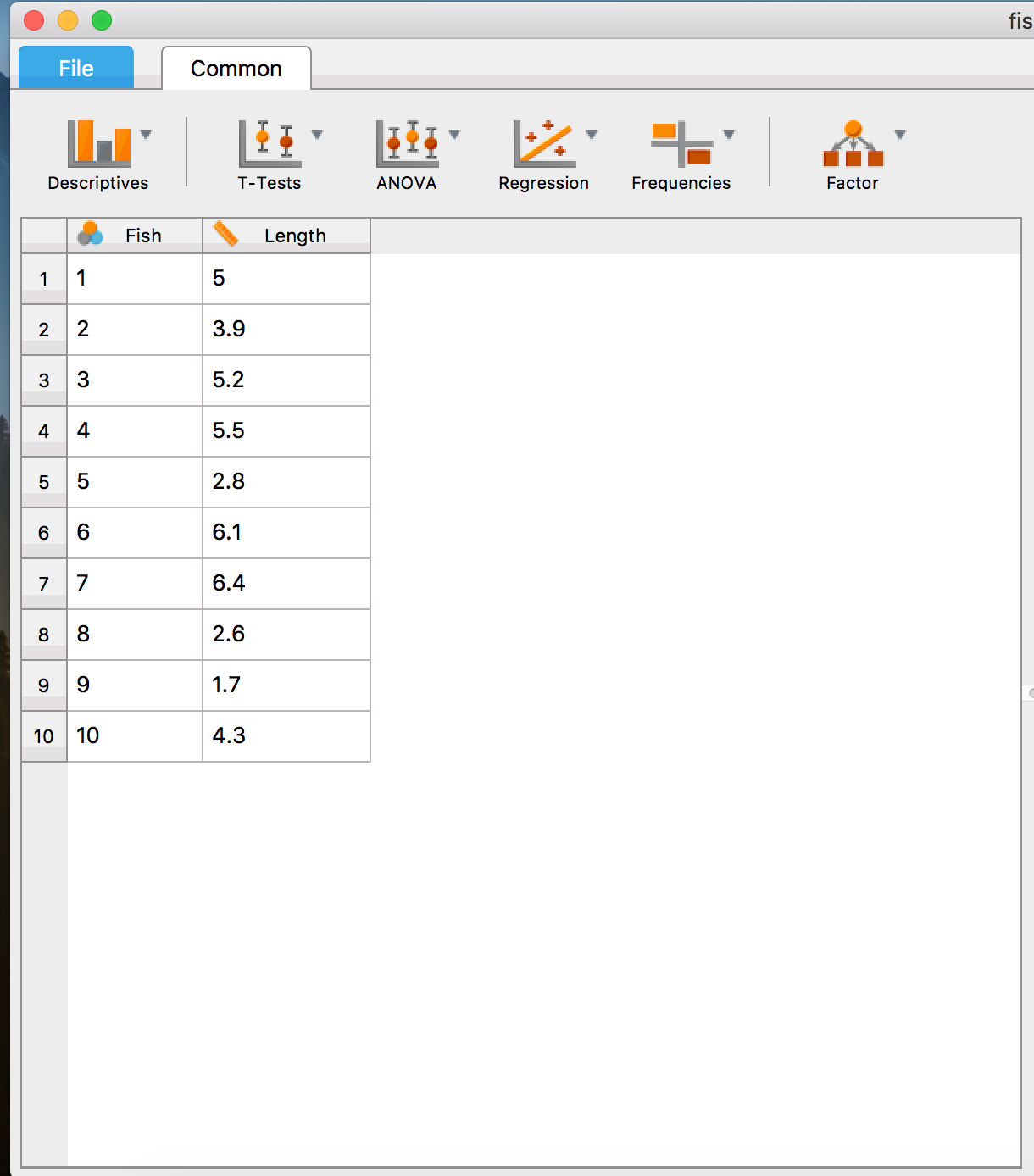#### Howdy, Stranger!

It looks like you're new here. If you want to get involved, click one of these buttons!

Supported by

# Wrong wording in t-test

When one wants to run independent samples T-test, there are three possible tests:
1. Student, when the distribution is normal and the two samples have equal variances
2. Welch, when the distribution is normal and the two samples don't have equal variances
3. Mann-Whitney U test, when the distribution is not normal

When one wants to run paired samples T-test, there are two possible tests:
1. Student, when the distribution is normal
2. Wilcoxon signed rank, when the distribution is not normal

When one wants to run one sample T-test, there are two possible tests:
1. Student, when the distribution is normal
2. "Mann-Whitney U test", when the distribution is not normal

i)However, this test is not "Mann-Whitney U test", but "one sample Wilcoxon signed rank test". U test is only for two independent samples. Check this website: https://onlinecourses.science.psu.edu/stat414/node/319
I inserted in JASP exactly the same numbers, I selected one-sample T Test, "Mann-Whitney U" test, as you have named it, and test value "3.7". JASP calculated a p-value=0.232, the same with the website. This means that JASP performs the same test as described in the website, but you have named the test incorrectly.
This might be confusing, so please correct it.

ii) Wilcoxon test uses median in the hypothesis, and not mean. This should be corrected (see screenshot).

iii) When you use the word "hypothesis", you should specify that this is the alternative hypothesis.• Hi Antonis,
Thanks a lot for taking the time to point this out. You are absolutely correct - the wording in the analysis form will be updated in the next JASP release. The underlying analysis, thankfully, does use the correct version of the Wilcoxon test (sign rank), hence its similarity to the online tool you used.
I will also update the hypothesis wording so that it is less confusing.
Cheers,
Johnny

• I see this post is from 2017 so hopefully improvements have been made. However, I have a problem with the Mann-Whitney in that it reports only the U and p values. Since it should function by comparing the mean ranks, I chose the option of descriptives under the M-W test - which provides only the mean, SD and SE values. Now I'm not sure what *exactly* JASP's M-W test is comparing - the means, the medians or mean ranks? And how can I get the mean ranks? Thanks :)

• Hi Majan,

What JASP reports for the Mann-Whitney test is the W statistic which is calculated by summing the ranks for one of the groups. In other words, when pooling the observations from both groups, and then assigning ranks, the W statistic is the sum of the ranks from either group. This value is then compared to the null-value to see if there are differences between the groups. This is the same as the wilcox.test() function in R. From their help-file:

"... the null hypothesis is that the distributions of x and y differ by a location shift of mu and the alternative is that they differ by some other location shift (and the one-sided alternative "greater" is that x is shifted to the right of y)."

As of now, JASP does not provide other descriptives of the rank-transformed observations. I agree that this is a nice additional feature to have - provide the mean rank of one group compared to another, for instance.

Kind regards,

Johnny

• Thanks for the reply! I'll see what to report and then leave the rest to the reviewers ;)